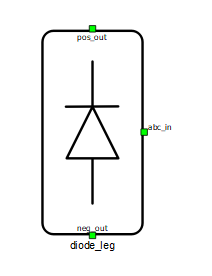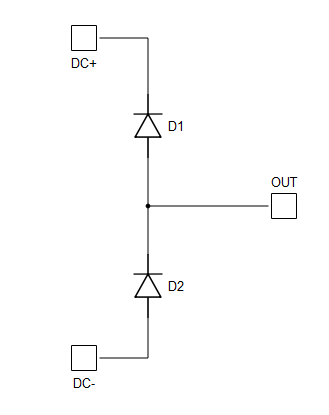# Diode leg

Description of the Diode Leg component in Schematic Editor

A block diagram and input parameters for the diode leg component are given in Table 1.

Table 1. A diode leg component in Schematic Editor core library
component component dialog window component parametersdiode leg• PESB _Optimization
Weight = 1

PESB Optimization, optimizes the switching block to reduce the memory requirements of the model.

The schematic block diagram of the diode leg block is given in Figure 1, with corresponding switch arrangement and naming.Figure 1. The schematic block diagram of a diode leg with corresponding switch naming

## Losses calculation

When the Losses calculation property is enabled, the component will calculate switching and conduction power losses for all switching elements (Diodes). Switching power losses are calculated as a function of current, voltage, and temperature using 3D Look-up tables (LUTs). Also, 2D input for losses is supported. When a 2D losses table is inserted, it assumes only current and temperature dependance. Conduction power losses can be defined as a function of current and temperature using Vd Look-up table.

Import options and an explanation of how to correctly fill in all the necessary power loss parameters are described in the Importing power losses data section.

Input/output power losses ports receive/generate vectors of two elements. The first element (index 0) is the upper diode, the second element (index 1) is the bottom diode.